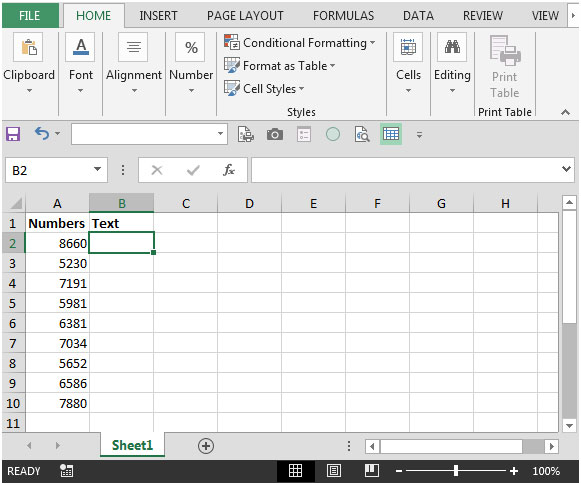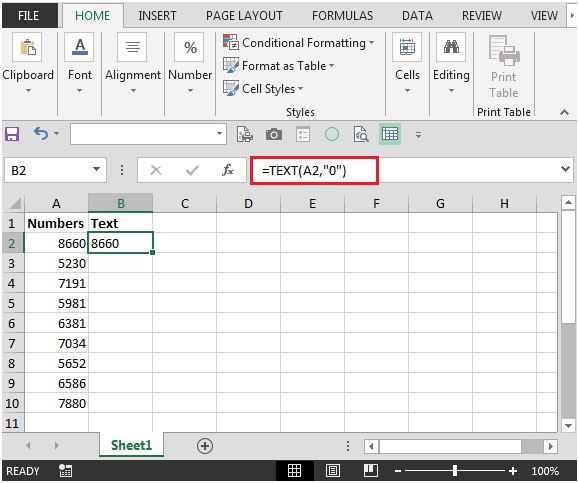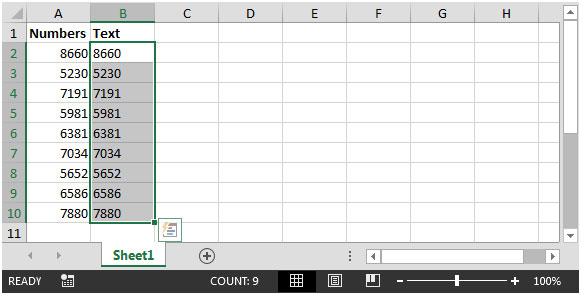# How to Convert Number to Text Using the Excel Text Function in Microsoft Excel 2010

If you want to convert number to text format, you can use TEXT function to achieve the output. It is one of the most used functions in Excel, providing flexibility the way you want to convert numbers to text. It converts a numeric value into text & allows you to specify the way the value will be displayed. This is a great help when you want to show numbers in readable format or if you want to join digits with text or symbols.

There is a negative side of using the TEXT function:it converts a numeric value to formatted text, hence the result cannot be calculated.

TEXT: Converts a value to text in a specific number format.

Syntax: =TEXT(value,format_text)

value: A numeric value that you want to be converted into text

format_text: Text string that defines the formatting that you want to be applied to the given value.

Let us understand with an example:

• We have a column that contains numbers• The formula in cell B2 would be =TEXT(A2,"0")• Copy the formula to below range of cells.To figure out the difference between the number & text in the alignment, you will find the numbers in column A are right aligned whereas numbers in column B are left aligned.

Terms and Conditions of use

The applications/code on this site are distributed as is and without warranties or liability. In no event shall the owner of the copyrights, or the authors of the applications/code be liable for any loss of profit, any problems or any damage resulting from the use or evaluation of the applications/code.